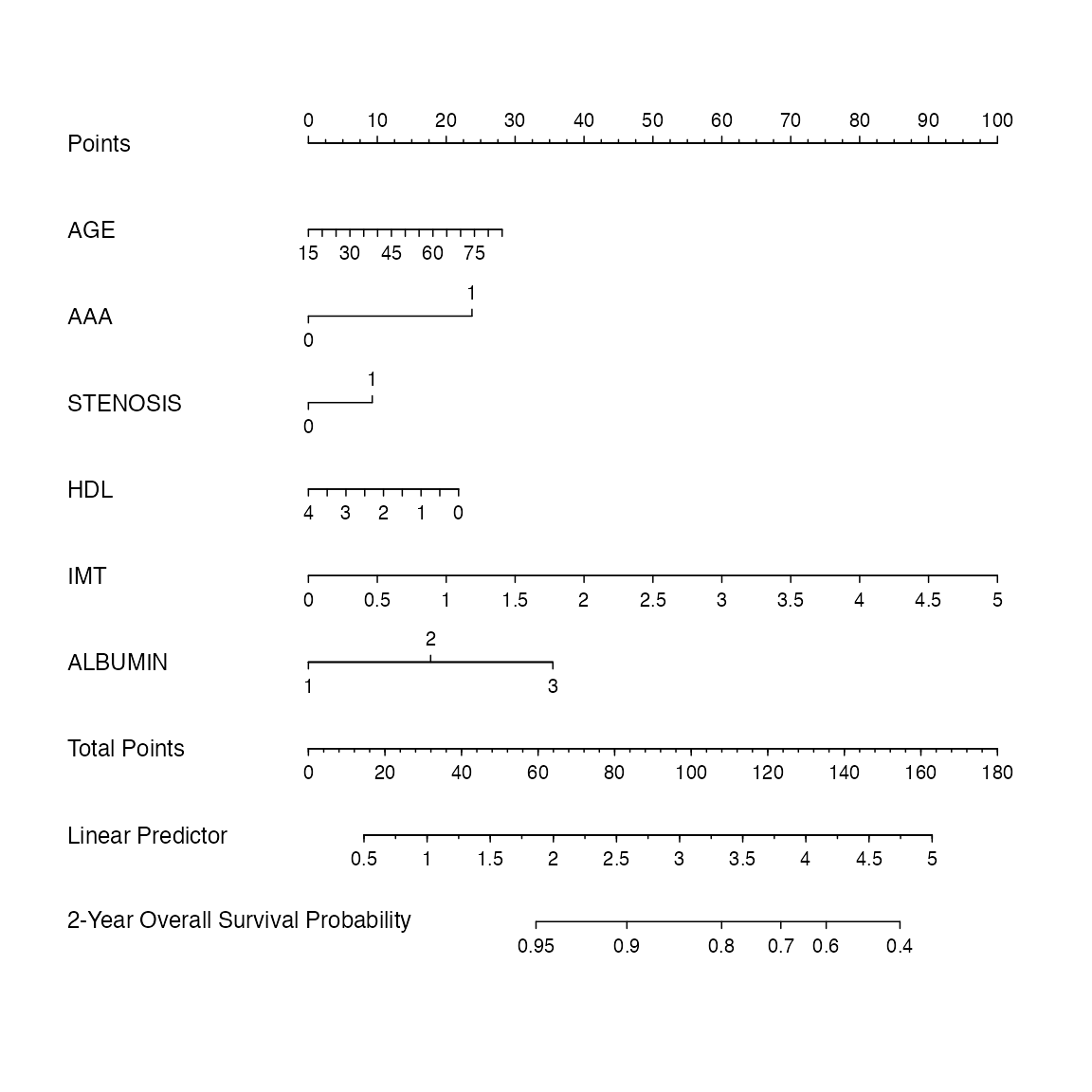## Introduction

It is a challenging task to model the emerging high-dimensional clinical data with survival outcomes. For its simplicity and efficiency, penalized Cox models are significantly useful for accomplishing such tasks.

hdnom streamlines the workflow of high-dimensional Cox model building, nomogram plotting, model validation, calibration, and comparison.

## Build survival models

To build a penalized Cox model with good predictive performance, some parameter tuning is usually needed. For example, the elastic-net model requires to tune the $$\ell_1$$-$$\ell_2$$ penalty trade-off parameter $$\alpha$$, and the regularization parameter $$\lambda$$.

To free the users from the tedious and error-prone parameter tuning process, hdnom provides several functions for automatic parameter tuning and model selection, including the following model types:

Function name Model type Auto-tuned hyperparameters
fit_lasso() Lasso $$\lambda$$
fit_alasso() Adaptive lasso $$\lambda$$
fit_enet() Elastic-net $$\lambda$$, $$\alpha$$
fit_aenet() Adaptive elastic-net $$\lambda$$, $$\alpha$$
fit_mcp() MCP $$\gamma$$, $$\lambda$$
fit_mnet() Mnet (MCP + $$\ell_2$$) $$\gamma$$, $$\lambda$$, $$\alpha$$
fit_scad() SCAD $$\gamma$$, $$\lambda$$
fit_snet() Snet (SCAD + $$\ell_2$$) $$\gamma$$, $$\lambda$$, $$\alpha$$
fit_flasso() Fused lasso $$\lambda_1$$, $$\lambda_2$$

In the next, we will use the imputed SMART study data to demonstrate a complete process of model building, nomogram plotting, model validation, calibration, and comparison with hdnom.

Load the packages and the smart dataset:

library("hdnom")
data("smart")
x <- as.matrix(smart[, -c(1, 2)])
time <- smart$TEVENT event <- smart$EVENT
y <- survival::Surv(time, event)

The dataset contains 3873 observations with corresponding survival outcome (time, event). 27 clinical variables (x) are available as the predictors. See ?smart for a detailed explanation of the variables.

Fit a penalized Cox model by adaptive elastic-net regularization with fit_aenet() and enable the parallel parameter tuning:

suppressMessages(library("doParallel"))
registerDoParallel(detectCores())

fit <- fit_aenet(x, y, nfolds = 10, rule = "lambda.1se", seed = c(5, 7), parallel = TRUE)
names(fit)
##  "model"       "alpha"       "lambda"      "model_init"  "alpha_init"
##  "lambda_init" "pen_factor"  "type"        "seed"        "call"  

Adaptive elastic-net includes two estimation steps. The random seed used for parameter tuning, the selected best $$\alpha$$, the selected best $$\lambda$$, the model fitted for each estimation step, and the penalty factor for the model coefficients in the second estimation step are all stored in the model object fit.

## Nomogram visualization

Before plotting the nomogram, we need to extract some necessary information about the model: the model object and the selected hyperparameters:

model <- fit$model alpha <- fit$alpha
lambda <- fit$lambda adapen <- fit$pen_factor

Let’s generate a nomogram object with as_nomogram() and plot it:

nom <- as_nomogram(
fit, x, time, event,
pred.at = 365 * 2,
funlabel = "2-Year Overall Survival Probability"
)

plot(nom)According to the nomogram, the adaptive elastic-net model selected 6 variables from the original set of 27 variables, effectively reduced the model complexity.

Information about the nomogram itself, such as the point-linear predictor unit mapping and total points-survival probability mapping, can be viewed by printing the nom object directly.

## Model validation

It is a common practice to utilize resampling-based methods to validate the predictive performance of a penalized Cox model. Bootstrap, $$k$$-fold cross-validation, and repeated $$k$$-fold cross-validation are the most employed methods for such purpose.

hdnom supports both internal model validation and external model validation. Internal validation takes the dataset used to build the model and evaluates the predictive performance on the data internally with the above resampling-based methods, while external validation evaluates the model’s predictive performance on a dataset which is independent to the dataset used in model building.

### Internal validation

validate() allows us to assess the model performance internally by time-dependent AUC (Area Under the ROC Curve) with the above three resampling methods.

Here, we validate the performance of the adaptive elastic-net model with bootstrap resampling, at every half year from the first year to the fifth year:

val_int <- validate(
x, time, event,
model.type = "aenet",
alpha = alpha, lambda = lambda, pen.factor = adapen,
method = "bootstrap", boot.times = 10,
tauc.type = "UNO", tauc.time = seq(1, 5, 0.5) * 365,
seed = 42, trace = FALSE
)

print(val_int)
#> High-Dimensional Cox Model Validation Object
#> Random seed: 42
#> Validation method: bootstrap
#> Bootstrap samples: 10
#> Model type: aenet
#> glmnet model alpha: 0.15
#> glmnet model lambda: 0.4322461
#> glmnet model penalty factor: specified
#> Time-dependent AUC type: UNO
#> Evaluation time points for tAUC: 365 547.5 730 912.5 1095 1277.5 1460 1642.5 1825
summary(val_int)
#> Time-Dependent AUC Summary at Evaluation Time Points
#>                365     547.5       730     912.5      1095    1277.5      1460
#> Mean     0.6736908 0.6980663 0.6883938 0.6877201 0.7171392 0.7329408 0.6801665
#> Min      0.6702972 0.6943275 0.6852397 0.6838341 0.7104875 0.7275005 0.6713858
#> 0.25 Qt. 0.6726018 0.6964805 0.6873354 0.6851884 0.7140499 0.7293569 0.6776290
#> Median   0.6740445 0.6983919 0.6879848 0.6884829 0.7163701 0.7321725 0.6799320
#> 0.75 Qt. 0.6749241 0.6996926 0.6896133 0.6896262 0.7210469 0.7371302 0.6825069
#> Max      0.6760826 0.7029078 0.6916850 0.6916987 0.7236970 0.7389818 0.6919914
#>             1642.5      1825
#> Mean     0.6841580 0.6935303
#> Min      0.6770945 0.6800316
#> 0.25 Qt. 0.6821133 0.6924729
#> Median   0.6831368 0.6956285
#> 0.75 Qt. 0.6864527 0.6966638
#> Max      0.6939574 0.6997908

The mean, median, 25%, and 75% quantiles of time-dependent AUC at each time point across all bootstrap predictions are listed above. The median and the mean can be considered as the bias-corrected estimation of the model performance.

It is also possible to plot the model validation result:

plot(val_int)
#>                365     547.5       730     912.5      1095    1277.5      1460
#> Mean     0.6736908 0.6980663 0.6883938 0.6877201 0.7171392 0.7329408 0.6801665
#> Min      0.6702972 0.6943275 0.6852397 0.6838341 0.7104875 0.7275005 0.6713858
#> 0.25 Qt. 0.6726018 0.6964805 0.6873354 0.6851884 0.7140499 0.7293569 0.6776290
#> Median   0.6740445 0.6983919 0.6879848 0.6884829 0.7163701 0.7321725 0.6799320
#> 0.75 Qt. 0.6749241 0.6996926 0.6896133 0.6896262 0.7210469 0.7371302 0.6825069
#> Max      0.6760826 0.7029078 0.6916850 0.6916987 0.7236970 0.7389818 0.6919914
#>             1642.5      1825
#> Mean     0.6841580 0.6935303
#> Min      0.6770945 0.6800316
#> 0.25 Qt. 0.6821133 0.6924729
#> Median   0.6831368 0.6956285
#> 0.75 Qt. 0.6864527 0.6966638
#> Max      0.6939574 0.6997908